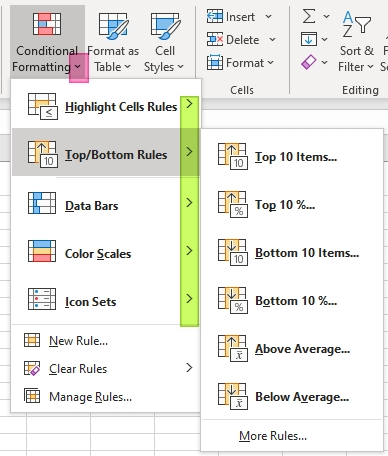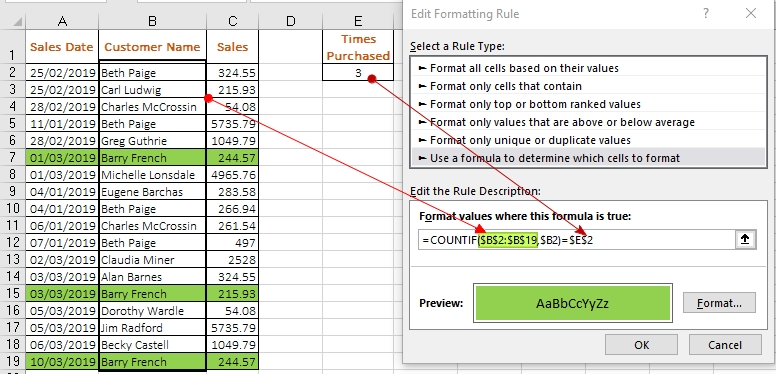#### Email Us

Follows

Learning how to use conditional formatting in excel can save you a lot of time when you need to visually highlight important information in a worksheet.

At a basic level, it can be used to highlight duplicates, values within certain thresholds, Top or Bottom N items, etc.However, to get the full potential of conditional formatting, you need to learn how to use formulas to highlight what you want. i.e create and manage rules

For example, how do you highlight triplicates, quadruplicates, etc–How do you highlight values repeated n times?

### Example 1

Highlight how many customers have been bought 3 times?Go to►Conditional Formatting►New Rule►Use a Formula to Determine which cell to format

Type below formula in the Formula bar ►Select your Format► Click Ok

`=COUNTIF(\$B\$2:\$B\$19,\$B2)=\$E\$2`

►Select the area to apply the format ►Apply ►Ok ► Close

### NB:

To highlight values repeated n times use below formula

`=COUNTIF(range,criteria)= n`

Highlighting a sample from a range is not any that tricky as long as you understand COUNTIF function and selecting the criteria.

i.e when selecting the criterion, select the first value in the column/row range and ensure you have fixed the column/row.

For example, how do you change the font color for sales 244.57,266.94,497?Go to►Conditional Formatting►New Rule►Use a Formula to Determine which cell to format. Insert below formula

`=COUNTIF(\$E\$2:\$E\$4,\$C2)=1`

### How It Works:

The formula counts how many times the sales (\$C2) appears in the sample List (\$E\$2:\$E\$4). If it appears 1 time, it returns a TRUE value which conditional format uses to highlight the cell.

What if you want to highlight sales between 2000 and 5000?`=COUNTIFS(\$C2, ">="&\$E\$2,  \$C2, "<="&\$E\$3)=1`

For this to work, you need to use the COUNTIFS function since you have to test for 2 criteria.

If the 2 criteria are met (equals to TRUE(1)), then condition format is applied.

### What if you want to highlight Sentences that contain certain words?

For example, how do you highlight sentences that contain either the word “excel” or “data” in the below sample?Go to►Conditional Formatting►New Rule►Use a Formula to Determine which cell to format. Insert below formula

`=SUM(COUNTIF(\$A2, "*"& \$C\$2:\$D\$2 &"*"))=1`

### How it Works

See this explanation from StackOverflow Forum

Introduction to Condition formating